x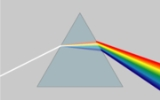Dispersion (optics)Encyclopedia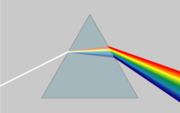In optics
Optics
Optics is the branch of physics which involves the behavior and properties of light, including its interactions with matter and the construction of instruments that use or detect it. Optics usually describes the behavior of visible, ultraviolet, and infrared light...

, dispersion is the phenomenon in which the phase velocity
Phase velocity
The phase velocity of a wave is the rate at which the phase of the wave propagates in space. This is the speed at which the phase of any one frequency component of the wave travels. For such a component, any given phase of the wave will appear to travel at the phase velocity...

of a wave depends on its frequency, or alternatively when the group velocity
Group velocity
The group velocity of a wave is the velocity with which the overall shape of the wave's amplitudes — known as the modulation or envelope of the wave — propagates through space....

depends on the frequency.
Media having such a property are termed dispersive media. Dispersion is sometimes called chromatic dispersion to emphasize its wavelength-dependent nature, or group-velocity dispersion (GVD) to emphasize the role of the group velocity.

The most familiar example of dispersion is probably a rainbow
Rainbow
A rainbow is an optical and meteorological phenomenon that causes a spectrum of light to appear in the sky when the Sun shines on to droplets of moisture in the Earth's atmosphere. It takes the form of a multicoloured arc...

, in which dispersion causes the spatial separation of a white light into components of different wavelengths (different color
Color
Color or colour is the visual perceptual property corresponding in humans to the categories called red, green, blue and others. Color derives from the spectrum of light interacting in the eye with the spectral sensitivities of the light receptors...

s). However, dispersion also has an effect in many other circumstances: for example, GVD causes pulses
Pulse (signal processing)
In signal processing, the term pulse has the following meanings:#A rapid, transient change in the amplitude of a signal from a baseline value to a higher or lower value, followed by a rapid return to the baseline value....

Optical fiber
An optical fiber is a flexible, transparent fiber made of a pure glass not much wider than a human hair. It functions as a waveguide, or "light pipe", to transmit light between the two ends of the fiber. The field of applied science and engineering concerned with the design and application of...

s, degrading signals over long distances; also, a cancellation between group-velocity dispersion and nonlinear effects leads to soliton
Soliton
In mathematics and physics, a soliton is a self-reinforcing solitary wave that maintains its shape while it travels at constant speed. Solitons are caused by a cancellation of nonlinear and dispersive effects in the medium...

waves. Dispersion is most often described for light
Light
Light or visible light is electromagnetic radiation that is visible to the human eye, and is responsible for the sense of sight. Visible light has wavelength in a range from about 380 nanometres to about 740 nm, with a frequency range of about 405 THz to 790 THz...

waves, but it may occur for any kind of wave that interacts with a medium or passes through an inhomogeneous geometry (e.g., a waveguide
Waveguide
A waveguide is a structure which guides waves, such as electromagnetic waves or sound waves. There are different types of waveguides for each type of wave...

), such as sound
Sound
Sound is a mechanical wave that is an oscillation of pressure transmitted through a solid, liquid, or gas, composed of frequencies within the range of hearing and of a level sufficiently strong to be heard, or the sensation stimulated in organs of hearing by such vibrations.-Propagation of...

waves.

There are generally two sources of dispersion: material dispersion and waveguide dispersion. Material dispersion comes from a frequency-dependent response of a material to waves. For example, material dispersion leads to undesired chromatic aberration
Chromatic aberration
In optics, chromatic aberration is a type of distortion in which there is a failure of a lens to focus all colors to the same convergence point. It occurs because lenses have a different refractive index for different wavelengths of light...

in a lens
Lens (optics)
A lens is an optical device with perfect or approximate axial symmetry which transmits and refracts light, converging or diverging the beam. A simple lens consists of a single optical element...

or the separation of colors in a prism
Dispersive prism
In optics, a dispersive prism is a type of optical prism, normally having the shape of a geometrical triangular prism. It is the most widely-known type of optical prism, although perhaps not the most common in actual use. Triangular prisms are used to disperse light, that is, to break light up into...

. Waveguide dispersion occurs when the speed of a wave in a waveguide (such as an optical fiber) depends on its frequency for geometric reasons, independent of any frequency dependence of the materials from which it is constructed. More generally, "waveguide" dispersion can occur for waves propagating through any inhomogeneous structure (e.g., a photonic crystal
Photonic crystal
Photonic crystals are periodic optical nanostructures that are designed to affect the motion of photons in a similar way that periodicity of a semiconductor crystal affects the motion of electrons...

), whether or not the waves are confined to some region. In general, both types of dispersion may be present, although they are not strictly additive. Their combination leads to signal degradation in optical fiber
Optical fiber
An optical fiber is a flexible, transparent fiber made of a pure glass not much wider than a human hair. It functions as a waveguide, or "light pipe", to transmit light between the two ends of the fiber. The field of applied science and engineering concerned with the design and application of...

s for telecommunication
Telecommunication
Telecommunication is the transmission of information over significant distances to communicate. In earlier times, telecommunications involved the use of visual signals, such as beacons, smoke signals, semaphore telegraphs, signal flags, and optical heliographs, or audio messages via coded...

s, because the varying delay in arrival time between different components of a signal "smears out" the signal in time.

## Material dispersion in optics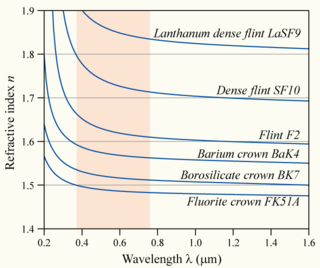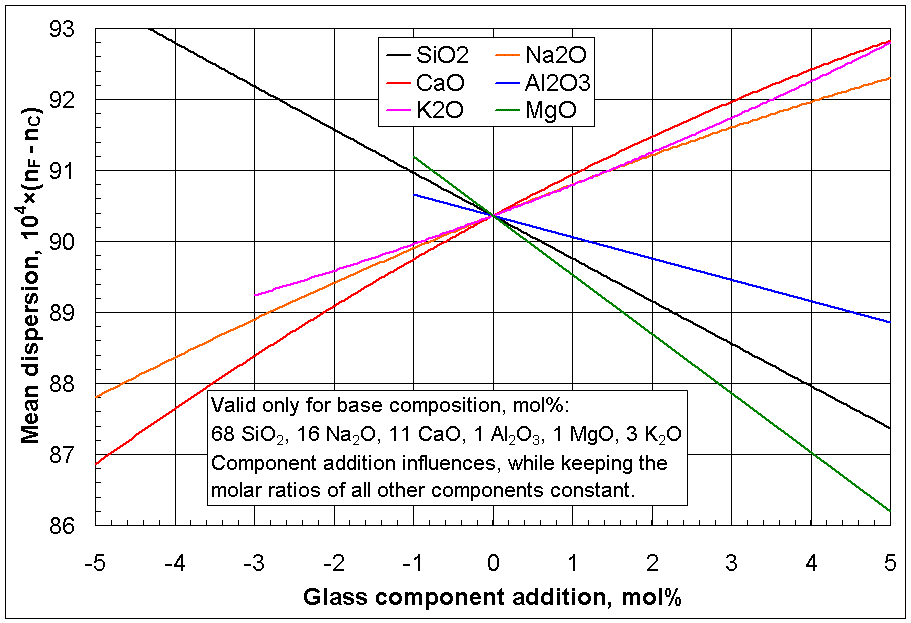Material dispersion can be a desirable or undesirable effect in optical applications. The dispersion of light by glass prisms is used to construct spectrometer
Spectrometer
A spectrometer is an instrument used to measure properties of light over a specific portion of the electromagnetic spectrum, typically used in spectroscopic analysis to identify materials. The variable measured is most often the light's intensity but could also, for instance, be the polarization...

Spectroradiometers are designed to measure the spectral power distributions of illuminants. They operate almost like spectrophotometers in the visible region...

s. Holographic gratings are also used, as they allow more accurate discrimination of wavelengths. However, in lenses, dispersion causes chromatic aberration
Chromatic aberration
In optics, chromatic aberration is a type of distortion in which there is a failure of a lens to focus all colors to the same convergence point. It occurs because lenses have a different refractive index for different wavelengths of light...

, an undesired effect that may degrade images in microscopes, telescopes and photographic objectives.

The phase velocity
Phase velocity
The phase velocity of a wave is the rate at which the phase of the wave propagates in space. This is the speed at which the phase of any one frequency component of the wave travels. For such a component, any given phase of the wave will appear to travel at the phase velocity...

, v, of a wave in a given uniform medium is given by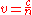where c is the speed of light
Speed of light
The speed of light in vacuum, usually denoted by c, is a physical constant important in many areas of physics. Its value is 299,792,458 metres per second, a figure that is exact since the length of the metre is defined from this constant and the international standard for time...

in a vacuum and n is the refractive index
Refractive index
In optics the refractive index or index of refraction of a substance or medium is a measure of the speed of light in that medium. It is expressed as a ratio of the speed of light in vacuum relative to that in the considered medium....

of the medium.

In general, the refractive index is some function of the frequency f of the light, thus n = n(f), or alternatively, with respect to the wave's wavelength n = n(λ). The wavelength dependence of a material's refractive index is usually quantified by its Abbe number
Abbe number
In physics and optics, the Abbe number, also known as the V-number or constringence of a transparent material, is a measure of the material's dispersion in relation to the refractive index...

or its coefficients in an empirical formula such as the Cauchy
Cauchy's equation
Cauchy's equation is an empirical relationship between the refractive index and wavelength of light for a particular transparent material. It is named for the mathematician Augustin Louis Cauchy, who defined it in 1836.-The equation:...

or Sellmeier equation
Sellmeier equation
The Sellmeier equation is an empirical relationship between refractive index and wavelength for a particular transparent medium. The equation is used to determine the dispersion of light in the medium....

s.

Because of the Kramers–Kronig relations, the wavelength dependence of the real part of the refractive index is related to the material absorption
In physics, absorption of electromagnetic radiation is the way by which the energy of a photon is taken up by matter, typically the electrons of an atom. Thus, the electromagnetic energy is transformed to other forms of energy for example, to heat. The absorption of light during wave propagation is...

, described by the imaginary part of the refractive index (also called the extinction coefficient). In particular, for non-magnetic materials (μ
Permeability (electromagnetism)
In electromagnetism, permeability is the measure of the ability of a material to support the formation of a magnetic field within itself. In other words, it is the degree of magnetization that a material obtains in response to an applied magnetic field. Magnetic permeability is typically...

= μ0), the susceptibility
Linear response function
A linear response function describes the input-output relationship of a signal transducer such as a radio turning electromagnetic waves into music or a neuron turning synaptic input into a response...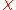that appears in the Kramers–Kronig relations is the electric susceptibility
Electric susceptibility
In electromagnetism, the electric susceptibility \chi_e is a dimensionless proportionality constant that indicates the degree of polarization of a dielectric material in response to an applied electric field...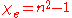.

The most commonly seen consequence of dispersion in optics is the separation of white light
White Light
White Light may refer to:*Light with the color white*White Light , a 1980 novel by Rudy Rucke*White Light , 1971 album*White Light , 2010 album...

into a color spectrum by a prism. From Snell's law
Snell's law
In optics and physics, Snell's law is a formula used to describe the relationship between the angles of incidence and refraction, when referring to light or other waves passing through a boundary between two different isotropic media, such as water and glass...

it can be seen that the angle of refraction
Refraction
Refraction is the change in direction of a wave due to a change in its speed. It is essentially a surface phenomenon . The phenomenon is mainly in governance to the law of conservation of energy. The proper explanation would be that due to change of medium, the phase velocity of the wave is changed...

of light in a prism depends on the refractive index of the prism material. Since that refractive index varies with wavelength, it follows that the angle that the light is refracted by will also vary with wavelength, causing an angular separation of the colors known as angular dispersion.

For visible light, refraction indices n of most transparent materials (e.g., air, glasses) decrease with increasing wavelength λ: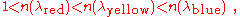or alternatively: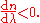In this case, the medium is said to have normal dispersion. Whereas, if the index increases with increasing wavelength (which is typically the case for X-ray
X-ray
X-radiation is a form of electromagnetic radiation. X-rays have a wavelength in the range of 0.01 to 10 nanometers, corresponding to frequencies in the range 30 petahertz to 30 exahertz and energies in the range 120 eV to 120 keV. They are shorter in wavelength than UV rays and longer than gamma...

s), the medium is said to have anomalous dispersion.

At the interface of such a material with air or vacuum (index of ~1), Snell's law predicts that light incident at an angle θ to the normal
Surface normal
A surface normal, or simply normal, to a flat surface is a vector that is perpendicular to that surface. A normal to a non-flat surface at a point P on the surface is a vector perpendicular to the tangent plane to that surface at P. The word "normal" is also used as an adjective: a line normal to a...

will be refracted at an angle arcsin(sin(θ)/n). Thus, blue light, with a higher refractive index, will be bent more strongly than red light, resulting in the well-known rainbow
Rainbow
A rainbow is an optical and meteorological phenomenon that causes a spectrum of light to appear in the sky when the Sun shines on to droplets of moisture in the Earth's atmosphere. It takes the form of a multicoloured arc...

pattern.

## Group and phase velocity

Another consequence of dispersion manifests itself as a temporal effect. The formula v = c / n calculates the phase velocity of a wave; this is the velocity
Velocity
In physics, velocity is speed in a given direction. Speed describes only how fast an object is moving, whereas velocity gives both the speed and direction of the object's motion. To have a constant velocity, an object must have a constant speed and motion in a constant direction. Constant ...

at which the phase
Phase (waves)
Phase in waves is the fraction of a wave cycle which has elapsed relative to an arbitrary point.-Formula:The phase of an oscillation or wave refers to a sinusoidal function such as the following:...

of any one frequency component of the wave will propagate. This is not the same as the group velocity
Group velocity
The group velocity of a wave is the velocity with which the overall shape of the wave's amplitudes — known as the modulation or envelope of the wave — propagates through space....

of the wave, that is the rate at which changes in amplitude
Amplitude
Amplitude is the magnitude of change in the oscillating variable with each oscillation within an oscillating system. For example, sound waves in air are oscillations in atmospheric pressure and their amplitudes are proportional to the change in pressure during one oscillation...

(known as the envelope of the wave) will propagate. For a homogeneous medium, the group velocity vg is related to the phase velocity by (here λ is the wavelength in vacuum, not in the medium):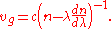The group velocity vg is often thought of as the velocity at which energy or information is conveyed along the wave. In most cases this is true, and the group velocity can be thought of as the signal velocity
Signal velocity
The signal velocity is the speed at which a wave carries information. It describes how quickly a message can be communicated between two separated parties...

of the waveform. In some unusual circumstances, called cases of anomalous dispersion, the rate of change of the index of refraction with respect to the wavelength changes sign, in which case it is possible for the group velocity to exceed the speed of light (vg > c). Anomalous dispersion occurs, for instance, where the wavelength of the light is close to an absorption resonance of the medium. When the dispersion is anomalous, however, group velocity is no longer an indicator of signal velocity. Instead, a signal travels at the speed of the wavefront, which is c irrespective of the index of refraction. Recently, it has become possible to create gases in which the group velocity is not only larger than the speed of light, but even negative. In these cases, a pulse can appear to exit a medium before it enters. Even in these cases, however, a signal travels at, or less than, the speed of light, as demonstrated by Stenner, et al.

The group velocity itself is usually a function of the wave's frequency. This results in group velocity dispersion (GVD), which causes a short pulse of light to spread in time as a result of different frequency components of the pulse travelling at different velocities. GVD is often quantified as the group delay dispersion parameter (again, this formula is for a uniform medium only):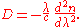If D is less than zero, the medium is said to have positive dispersion. If D is greater than zero, the medium has negative dispersion. If a light pulse is propagated through a normally dispersive medium, the result is the higher frequency components travel slower than the lower frequency components. The pulse therefore becomes positively chirp
Chirp
A chirp is a signal in which the frequency increases or decreases with time. In some sources, the term chirp is used interchangeably with sweep signal. It is commonly used in sonar and radar, but has other applications, such as in spread spectrum communications...

ed, or up-chirped, increasing in frequency with time. Conversely, if a pulse travels through an anomalously dispersive medium, high frequency components travel faster than the lower ones, and the pulse becomes negatively chirp
Chirp
A chirp is a signal in which the frequency increases or decreases with time. In some sources, the term chirp is used interchangeably with sweep signal. It is commonly used in sonar and radar, but has other applications, such as in spread spectrum communications...

ed, or down-chirped, decreasing in frequency with time.

The result of GVD, whether negative or positive, is ultimately temporal spreading of the pulse. This makes dispersion management extremely important in optical communications systems based on optical fiber, since if dispersion is too high, a group of pulses representing a bit-stream will spread in time and merge together, rendering the bit-stream unintelligible. This limits the length of fiber that a signal can be sent down without regeneration. One possible answer to this problem is to send signals down the optical fibre at a wavelength where the GVD is zero (e.g., around 1.3–1.5 μm in silica fibres), so pulses at this wavelength suffer minimal spreading from dispersion—in practice, however, this approach causes more problems than it solves because zero GVD unacceptably amplifies other nonlinear effects (such as four wave mixing). Another possible option is to use soliton
Soliton (optics)
In optics, the term soliton is used to refer to any optical field that does not change during propagation because of a delicate balance between nonlinear and linear effects in the medium. There are two main kinds of solitons:...

pulses in the regime of anomalous dispersion, a form of optical pulse which uses a nonlinear optical
Nonlinear optics
Nonlinear optics is the branch of optics that describes the behavior of light in nonlinear media, that is, media in which the dielectric polarization P responds nonlinearly to the electric field E of the light...

effect to self-maintain its shape—solitons have the practical problem, however, that they require a certain power level to be maintained in the pulse for the nonlinear effect to be of the correct strength. Instead, the solution that is currently used in practice is to perform dispersion compensation, typically by matching the fiber with another fiber of opposite-sign dispersion so that the dispersion effects cancel; such compensation is ultimately limited by nonlinear effects such as self-phase modulation
Self-phase modulation
Self-phase modulation is a nonlinear optical effect of light-matter interaction.An ultrashort pulse of light, when travelling in a medium, will induce a varying refractive index of the medium due to the optical Kerr effect...

, which interact with dispersion to make it very difficult to undo.

Dispersion control is also important in laser
Laser
A laser is a device that emits light through a process of optical amplification based on the stimulated emission of photons. The term "laser" originated as an acronym for Light Amplification by Stimulated Emission of Radiation...

s that produce short pulses
Ultrashort pulse
In optics, an ultrashort pulse of light is an electromagnetic pulse whose time duration is of the order of a femtosecond . Such pulses have a broadband optical spectrum, and can be created by mode-locked oscillators...

. The overall dispersion of the optical resonator
Laser construction
A laser is constructed from three principal parts:*An energy source ,*A gain medium or laser medium, and*Two or more mirrors that form an optical resonator.-Pump source:...

is a major factor in determining the duration of the pulses emitted by the laser. A pair of prism
Prism (optics)
In optics, a prism is a transparent optical element with flat, polished surfaces that refract light. The exact angles between the surfaces depend on the application. The traditional geometrical shape is that of a triangular prism with a triangular base and rectangular sides, and in colloquial use...

s can be arranged to produce net negative dispersion, which can be used to balance the usually positive dispersion of the laser medium. Diffraction grating
Diffraction grating
In optics, a diffraction grating is an optical component with a periodic structure, which splits and diffracts light into several beams travelling in different directions. The directions of these beams depend on the spacing of the grating and the wavelength of the light so that the grating acts as...

s can also be used to produce dispersive effects; these are often used in high-power laser amplifier systems. Recently, an alternative to prisms and gratings has been developed: chirped mirror
Chirped mirror
A chirped mirror is a dielectric mirror with chirped spaces—spaces of varying depth designed to reflect varying wavelengths of lights—between the dielectric layers ....

s. These dielectric mirrors are coated so that different wavelengths have different penetration lengths, and therefore different group delays. The coating layers can be tailored to achieve a net negative dispersion.

## Dispersion in waveguides

Optical fibers, which are used in telecommunications, are among the most abundant types of waveguides. Dispersion in these fibers is one of the limiting factors that determine how much data can be transported on a single fiber.

The transverse mode
Transverse mode
A transverse mode of a beam of electromagnetic radiation is a particular electromagnetic field pattern of radiation measured in a plane perpendicular to the propagation direction of the beam...

s for waves confined laterally within a waveguide
Waveguide
A waveguide is a structure which guides waves, such as electromagnetic waves or sound waves. There are different types of waveguides for each type of wave...

generally have different speeds (and field patterns) depending upon their frequency (that is, on the relative size of the wave, the wavelength) compared to the size of the waveguide.

In general, for a waveguide mode with an angular frequency
Angular frequency
In physics, angular frequency ω is a scalar measure of rotation rate. Angular frequency is the magnitude of the vector quantity angular velocity...

ω(β) at a propagation constant
Propagation constant
The propagation constant of an electromagnetic wave is a measure of the change undergone by the amplitude of the wave as it propagates in a given direction. The quantity being measured can be the voltage or current in a circuit or a field vector such as electric field strength or flux density...

β (so that the electromagnetic fields in the propagation direction (z) oscillate proportional to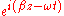), the group-velocity dispersion parameter D is defined as: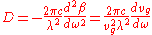where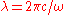is the vacuum wavelength and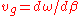is the group velocity. This formula generalizes the one in the previous section for homogeneous media, and includes both waveguide dispersion and material dispersion. The reason for defining the dispersion in this way is that |D| is the (asymptotic) temporal pulse spreading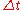per unit bandwidth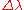per unit distance travelled, commonly reported in ps
Picosecond
A picosecond is 10−12 of a second. That is one trillionth, or one millionth of one millionth of a second, or 0.000 000 000 001 seconds. A picosecond is to one second as one second is to 31,700 years....

/ nm
Nanometre
A nanometre is a unit of length in the metric system, equal to one billionth of a metre. The name combines the SI prefix nano- with the parent unit name metre .The nanometre is often used to express dimensions on the atomic scale: the diameter...

km for optical fibers.

A similar effect due to a somewhat different phenomenon is modal dispersion
Modal dispersion
Modal dispersion is a distortion mechanism occurring in multimode fibers and other waveguides, in which the signal is spread in time because the propagation velocity of the optical signal is not the same for all modes...

, caused by a waveguide having multiple modes at a given frequency, each with a different speed. A special case of this is polarization mode dispersion
Polarization mode dispersion
Polarization mode dispersion is a form of modal dispersion where two different polarizations of light in a waveguide, which normally travel at the same speed, travel at different speeds due to random imperfections and asymmetries, causing random spreading of optical pulses...

(PMD), which comes from a superposition of two modes that travel at different speeds due to random imperfections that break the symmetry of the waveguide.

## Higher-order dispersion over broad bandwidths

When a broad range of frequencies (a broad bandwidth) is present in a single wavepacket, such as in an ultrashort pulse
Ultrashort pulse
In optics, an ultrashort pulse of light is an electromagnetic pulse whose time duration is of the order of a femtosecond . Such pulses have a broadband optical spectrum, and can be created by mode-locked oscillators...

or a chirp
Chirp
A chirp is a signal in which the frequency increases or decreases with time. In some sources, the term chirp is used interchangeably with sweep signal. It is commonly used in sonar and radar, but has other applications, such as in spread spectrum communications...

ed pulse or other forms of spread spectrum
Spread-spectrum techniques are methods by which a signal generated in a particular bandwidth is deliberately spread in the frequency domain, resulting in a signal with a wider bandwidth...

transmission, it may not be accurate to approximate the dispersion by a constant over the entire bandwidth, and more complex calculations are required to compute effects such as pulse spreading.

In particular, the dispersion parameter D defined above is obtained from only one derivative of the group velocity. Higher derivatives are known as higher-order dispersion. These terms are simply a Taylor series
Taylor series
In mathematics, a Taylor series is a representation of a function as an infinite sum of terms that are calculated from the values of the function's derivatives at a single point....

expansion of the dispersion relation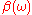of the medium or waveguide around some particular frequency. Their effects can be computed via numerical evaluation of Fourier transform
Fourier transform
In mathematics, Fourier analysis is a subject area which grew from the study of Fourier series. The subject began with the study of the way general functions may be represented by sums of simpler trigonometric functions...

s of the waveform, via integration of higher-order slowly varying envelope approximation
Slowly varying envelope approximation
In physics, the slowly varying envelope approximation is the assumption that the envelope of a forward-travelling wave pulse varies slowly in time and space compared to a period or wavelength...

s, by a split-step method
Split-step method
In numerical analysis, the split-step method is a pseudo-spectral numerical method used to solve nonlinear partial differential equations like the nonlinear Schrödinger equation. The name arises for two reasons. First, the method relies on computing the solution in small steps, and treating the...

(which can use the exact dispersion relation rather than a Taylor series), or by direct simulation of the full Maxwell's equations
Maxwell's equations
Maxwell's equations are a set of partial differential equations that, together with the Lorentz force law, form the foundation of classical electrodynamics, classical optics, and electric circuits. These fields in turn underlie modern electrical and communications technologies.Maxwell's equations...

rather than an approximate envelope equation.

## Dispersion in gemology

In the technical terminology
Technical terminology
Technical terminology is the specialized vocabulary of any field, not just technical fields. The same is true of the synonyms technical terms, terms of art, shop talk and words of art, which do not necessarily refer to technology or art...

of gemology
Gemology
Gemology or gemmology is the science dealing with natural and artificial gems and gemstones. It is considered a geoscience and a branch of mineralogy...

, dispersion is the difference in the refractive index of a material at the B and G Fraunhofer wavelengths of 686.7 nm
Nanometre
A nanometre is a unit of length in the metric system, equal to one billionth of a metre. The name combines the SI prefix nano- with the parent unit name metre .The nanometre is often used to express dimensions on the atomic scale: the diameter...

and 430.8 nm and is meant to express the degree to which a prism cut from the gemstone
Gemstone
A gemstone or gem is a piece of mineral, which, in cut and polished form, is used to make jewelry or other adornments...

shows "fire", or color. Dispersion is a material property. Fire depends on the dispersion, the cut angles, the lighting environment, the refractive index, and the viewer.

## Dispersion in imaging

In photographic and microscopic lenses, dispersion causes chromatic aberration
Chromatic aberration
In optics, chromatic aberration is a type of distortion in which there is a failure of a lens to focus all colors to the same convergence point. It occurs because lenses have a different refractive index for different wavelengths of light...

, which causes the different colors in the image not to overlap properly. Various techniques have been developed to counteract this, such as the use of achromats, multielement lenses with glasses of different dispersion. They are constructed in such a way that the chromatic aberrations of the different parts cancel out.

## Dispersion in pulsar timing

Pulsar
Pulsar
A pulsar is a highly magnetized, rotating neutron star that emits a beam of electromagnetic radiation. The radiation can only be observed when the beam of emission is pointing towards the Earth. This is called the lighthouse effect and gives rise to the pulsed nature that gives pulsars their name...

s are spinning neutron stars that emit pulses at very regular intervals ranging from milliseconds to seconds. Astronomers believe that the pulses are emitted simultaneously over a wide range of frequencies. However, as observed on Earth, the components of each pulse emitted at higher radio frequencies arrive before those emitted at lower frequencies. This dispersion occurs because of the ionised component of the interstellar medium
Interstellar medium
In astronomy, the interstellar medium is the matter that exists in the space between the star systems in a galaxy. This matter includes gas in ionic, atomic, and molecular form, dust, and cosmic rays. It fills interstellar space and blends smoothly into the surrounding intergalactic space...

, which makes the group velocity frequency dependent. The extra delay added at a frequencyis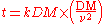where the dispersion constant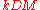is given by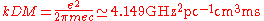,

and the dispersion measure DM is the free electron column density (total electron content
Total electron content
Total electron content is an important descriptive quantity for the ionosphere of the Earth. TEC is the total number of electrons present along a path between two points, with units of electrons per square meter, where 1016 electrons/m² = 1 TEC unit .TEC is significant in determining the...

)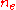integrated along the path traveled by the photon from the pulsar to the Earth, and is given by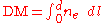with units of parsec
Parsec
The parsec is a unit of length used in astronomy. It is about 3.26 light-years, or just under 31 trillion kilometres ....

s per cubic centimetre (1pc/cm3 = 30.857×1021 m−2).

Typically for astronometric observations, this delay cannot be measured directly, since the emission time is unknown. What can be measured is the difference in arrival times at two different frequencies. The delay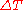between a high frequency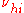and a low frequency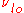component of a pulse will be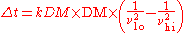Re-writing the above equation in terms of DM allows one to determine the DM by measuring pulse arrival times at multiple frequencies. This in turn can be used to study the interstellar medium, as well as allow for observations of pulsars at different frequencies to be combined.

• Dispersion relation
Dispersion relation
In physics and electrical engineering, dispersion most often refers to frequency-dependent effects in wave propagation. Note, however, that there are several other uses of the word "dispersion" in the physical sciences....

• Sellmeier equation
Sellmeier equation
The Sellmeier equation is an empirical relationship between refractive index and wavelength for a particular transparent medium. The equation is used to determine the dispersion of light in the medium....

• Cauchy's equation
Cauchy's equation
Cauchy's equation is an empirical relationship between the refractive index and wavelength of light for a particular transparent material. It is named for the mathematician Augustin Louis Cauchy, who defined it in 1836.-The equation:...

• Abbe number
Abbe number
In physics and optics, the Abbe number, also known as the V-number or constringence of a transparent material, is a measure of the material's dispersion in relation to the refractive index...

• Kramers–Kronig relation
• Group delay
Group delay
Group delay is a measure of the time delay of the amplitude envelopes of the various sinusoidal components of a signal through a device under test, and is a function of frequency for each component...

• Calculation of glass properties
Calculation of glass properties
The calculation of glass properties is used to predict glass properties of interest or glass behavior under certain conditions without experimental investigation, based on past data and experience, with the intention to save time, material, financial, and environmental resources, or to gain...

incl. dispersion
• Linear response function
Linear response function
A linear response function describes the input-output relationship of a signal transducer such as a radio turning electromagnetic waves into music or a neuron turning synaptic input into a response...

• Green-Kubo relations
Green-Kubo relations
The Green–Kubo relations give the exact mathematical expression for transport coefficients in terms of integrals of time correlation functions.-Thermal and mechanical transport processes:...

• Fluctuation theorem
Fluctuation theorem
The fluctuation theorem , which originated from statistical mechanics, deals with the relative probability that the entropy of a system which is currently away from thermodynamic equilibrium will increase or decrease over a given amount of time...

• Multiple-prism dispersion theory
Multiple-prism dispersion theory
The first description of multiple-prism arrays, and multiple-prism dispersion, was given by Newton in his book Opticks. Prism pair expanders were introduced by Brewster in 1813. A modern mathematical description of the single-prism dispersion was given by Born and Wolf in 1959...

• Ultrashort pulse
Ultrashort pulse
In optics, an ultrashort pulse of light is an electromagnetic pulse whose time duration is of the order of a femtosecond . Such pulses have a broadband optical spectrum, and can be created by mode-locked oscillators...